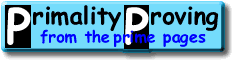Finding primes & proving primality 4.3: A Polynomial-Time AlgorithmHome > Primality Proving > Chapter Four > A Polynomial-Time Algorithm

As we mentioned before, many of the primality proving methods are conjectured to be polynomial-time.  For example, Miller's test is polynomial if ERH is true (and Rabin gave a version of this test that was unconditionally randomized polynomial-time [Rabin80]).  Adleman and Hang [AH1992] modified the Goldwasser-Killian algorithm [GK86] to produce a randomized polynomial time algorithm that always produced a certificate of primality...  So it is not surprising that there exists a polynomial-time algorithm for proving primality.  But what is surprising is that in 2002 Agrawal, Kayal and Saxena [AKS2002] found a relatively simple deterministic algorithm which relies on no unproved assumptions.  We present this algorithm below then briefly refer to a related algorithm of Bernstein.

The key to AKS' result is another simple version of Fermat's Little Theorem:

Theorem:  Suppose that a and p are relatively prime integers with p > 1.  p is prime if and only if

(x-a)p ≡ (xp-a)   (mod p)
Proof.  If p is prime, then p divides the binomial coefficients pCr for r = 1, 2, ... p-1.  This shows that (x-a)p ≡ (xp-ap) (mod p), and the equation above follows via Fermat's Little Theorem.  On the other hand, if p > 1 is composite, then it has a prime divisor q.  Let qk be the greatest power of q that divides p. Then qk does not divide pCq and is relatively prime to ap-q, so the coefficient of the term xq on the left of the equation in the theorem is not zero, but it is on the right.

(This result was used to create a randomized polynomial-time algorithm by Agrawal and Biswas [AB1999].)

Of course in this form it is too difficult to use because there are just far too many coefficients to check.  Their idea was to look at the simpler condition:

(x-a)p ≡ (xp-a)   (mod xr-1,p)

This must hold if p is prime and it is conjectured (see [BP2001, KS2002]) that if r >1 does not divide p and the above congruence holds, then either p is prime or p2 is 1 modulo r.

Agrawal, Kayal and Saxena managed to reformulate this into the following algorithm which they proved would run in at most O((log n)12f(log log n)) time where f is a polynomial. (This means the time it takes to run the algorithm is at most a constant times the number of digits to the twelfth power times a polynomial evaluated at the log of the number of digits.)

```Input: Integer n > 1 if (n is has the form ab with b > 1) then output COMPOSITE r := 2 while (r < n) {     if (gcd(n,r) is not 1) then output COMPOSITE     if (r is prime greater than 2) then {         let q be the largest factor of r-1         if (q > 4sqrt(r)log n) and (n(r-1)/q is not 1 (mod r)) then break     }     r := r+1 } for a = 1 to 2sqrt(r)log n {     if ( (x-a)n is not (xn-a) (mod xr-1,n) ) then output COMPOSITE } output PRIME;```

The proof [AKS2002] is relatively straightforward, and perhaps the most advanced result necessary is a sieve result required to show the necessary q exists for each composite ([F1985], [BH1996]).  (Note that the first step, determining if the number is a perfect power, can be done in essentially linear time [Bernstein1998b].)

AKS also showed that if Sophie Germain primes have the expected distribution [HL23] (and they certainly should!), then the exponent 12 in the time estimate can be reduced to 6, bringing it much closer to the (probabilistic) ECPP method.  But of course when actually finding primes it is the unlisted constants1 that make all of the difference!  We will have to wait for efficient implementations of this algorithm (and hopefully clever restatements of the painful` for loop`) to see how it compares to the others for integers of a few thousand digits.  Until then, at least we have learned that there is a polynomial-time algorithm for all integers that both is deterministic and relies on no unproved conjectures!

Note: D. J. Bernstein's exposition of the Agrawal-Kayal-Saxena theorem (mentioned above) contains improvements by many diferent researchers which reduce the constants involved in the time analysis by at least a factor of 2,000,000.  This is perhaps the best source for the present state of the algorithm.

### Related Approaches

Berrizbeitia [Berrizbeitia2003] found a way to save time in AKS-type primality proofs for some primes n, reducing the exponent from 6+o(1) to 4+o(1).  Cheng [Cheng2003] extended Berrizbeitia's idea to more primes n, and Bernstein [Bernstein2003] extended it to all primes n.  The algorithm for finding these proofs relies on some randomness, unlike the original AKS algorithm.

It seems plausible that a variant of AKS may soon compete in practice with ECPP for 'general' primality proofs.  This field is in great deal of flux.